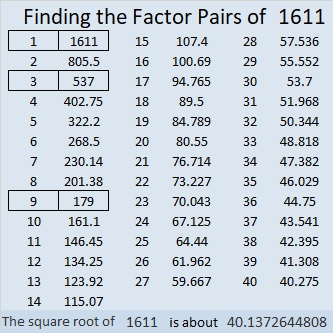# 1611 A Little Blarney?

### Today’s Puzzle:

Many travelers to Ireland hang upsidedown to kiss the Blarney Stone at the top of Blarney castle. There is even a Sherlock Holmes mystery about someone who appears to have fallen to his death while trying to kiss the Blarney Stone. If I said this mystery level puzzle represents the Blarney Stone, would that just be a bunch of blarney?Print the puzzles or type the solution in this excel file: 14 Factors 1604-1612.

### Factors of 1611:

• 1611 is a composite number.
• Prime factorization: 1611 = 3 × 3 × 179, which can be written 1611 = 3² × 179.
• 1611 has at least one exponent greater than 1 in its prime factorization so √1611 can be simplified. Taking the factor pair from the factor pair table below with the largest square number factor, we get √1611 = (√9)(√179) = 3√179.
• The exponents in the prime factorization are 2 and 1. Adding one to each exponent and multiplying we get (2 + 1)(1 + 1) = 3 × 2 = 6. Therefore 1611 has exactly 6 factors.
• The factors of 1611 are outlined with their factor pair partners in the graphic below.### More about the number 1611:

1611 is the difference of two squares in three different ways:
806² – 805² = 1611,
270² – 267² = 1611, and
94² – 85² = 1611.